# High School Chemistry : Titrations

## Example Questions

← Previous 1 3 4

### Example Question #1 : Identifying Unknown Concentration

A 100mL solution is composed of 25% ethanol by volume and water. What is the mass of the solution?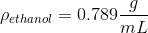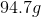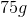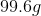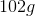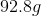Explanation:

First we determine the mass of the ethanol in solution using its density. Using the percent by volume of ethanol, we know that there are 25mL of ethanol in a 100mL solution. The remaining 75mL are water.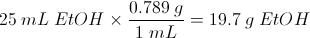Since the density of water is 1g/mL, we know that the mass of 75mL of water is 75g. The total mass is the sum of the ethanol and the water.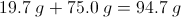### Example Question #2 : Identifying Unknown Concentration

What is the concentration if 40 g NaOH is dissolved in 1000 g of water

1 m
4 m
4 M
40 M
1 M
Explanation:

first of all, M = molar; m = molal- M = mol solute/ L of solution; m = mol solute/ kg solvent

you have 40 g NaOH * 1 mol/40 g = 1 mol

1000 g of water is equivalent to 1 L

1 mol/L = 1M

### Example Question #1 : Identifying Unknown Concentration

80.0g NaOH is put into 50000 mL water.  What is the molarity of the resulting solution?

1.6 M

0.625 M

0.04 M

0.00004 M

0.0016 M

0.04 M

Explanation:

Molarity = mol solute / L soution

mol solute = 80 g NaOH * 1 mol / 40 g = 2 mol

L solution = 50000 mL water * 1 L/1000 mL = 50 L

2 mol / 50 L =

### Example Question #4 : Identifying Unknown Concentration

How many liters of 10M HCl are needed to make 4L of 0.4M solution?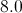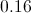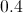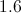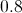Explanation:

A simple calculation can be done to perform any solution dilution problem. We know our equation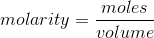.

We can rewrite this as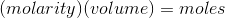.

Using this formula, we take the old solution and set it equal to the new solution.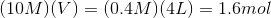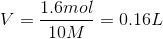We need 0.16 liters of our 10 molar solution.

### Example Question #5 : Identifying Unknown Concentration

Which solution has the highest molarity?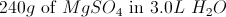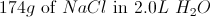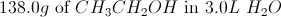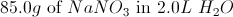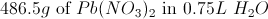Explanation:

This question requires us to calculate molarity for each answer choice. It is important to add everything correctly and be careful with more complex compounds.

Molarity is simply moles of solute over liters of solution. The correct answer, after trying each, is the answer with lead (II) nitrate, as it gets us a molarity of 2.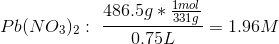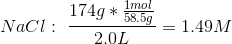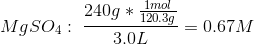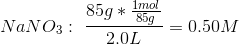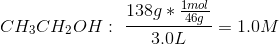### Example Question #6 : Identifying Unknown Concentration

Which of the following aqueous solutions is the most concentrated?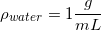All of these solutions have the same concentration.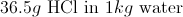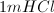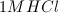Explanation:

In order to answer this question, it helps to know that 1 kilogram of water is equal to 1 liter of water, due to its density. Two of the above options refer to a 1m solution of hydrochloric acid. The other is a 1M solution.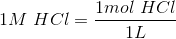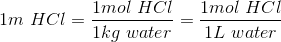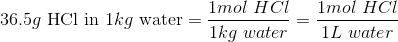All three of the options have the same amount of hydrochloric acid (one mole). For molarity, the hydrochloric acid is diluted with water until one liter of solution is created. For molality, one mole of HCl is added to one kilogram of water. Since one kilogram of water is one liter, this becomes the same concentration.

One a very small level, the 1M HCl solution will be slightly more concentrated. Creating a molal solution does not take into account the volume of the solute. If, for example, 100 cubic centimeters of HCl were added to one kilogram of water, the resulting volume would be more than one liter, making the concentration slightly less than 1M. This discrepancy is usually not accounted for in basic chemistry, but you should be familiar with the concept.

### Example Question #1 : Identifying Unknown Concentration

What is the molality of a solution created by mixing 4.3g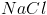into 43g of water?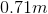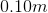Cannot be determined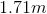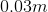Explanation:

Molality can be defined: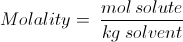It is slightly different from Molarity and has different uses.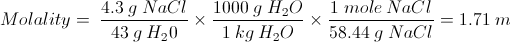### Example Question #8 : Identifying Unknown Concentration

How much solid NaOH must be dissolved to make 740mL of a 0.32M solution?

9.47 * 102g

4.26g

12.8g

9.47g

The sodium hydroxide will boil off with the water

9.47g

Explanation:

This problem can be solved by stoichiometry. Remember that 0.32M gives us the moles of NaOH per liter, and solve for the number of moles per 0.740L.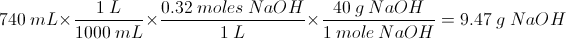### Example Question #9 : Identifying Unknown Concentration

Find the mass of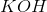in 10L of water if it is a 2m solution.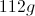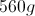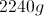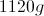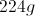Explanation:

Molality is grams of solute per kilogram of solvent.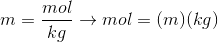Water has a density of one gram per mililiter, so one liter of water equal to one kilogram. If we have a 2m solution, that means we have two moles ofper kilogram of water.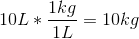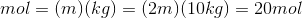has a molecular weight of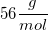.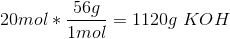This gives us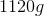of.

### Example Question #10 : Identifying Unknown Concentration

10mL of a solution of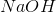of unknown concentration mixed with 34mL of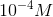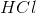produced a solution with a pH of 7. What is the concentration of thesolution?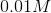None of these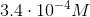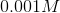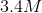Explanation:

For this question use the following formula: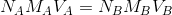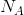is the number of acidic hydrogens on the acid,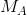is the molarity of the acid,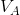is the volume of the acid,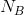is the number of basic hydroxides on the base,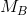is the molarity of the base,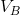is the volume of the base

Rearrange the equation for the molarity of the base: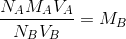Plug in known values and solve.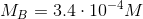← Previous 1 3 4

### All High School Chemistry Resources